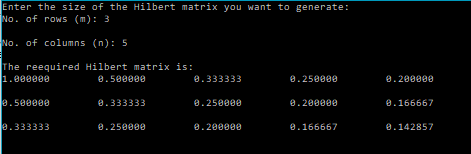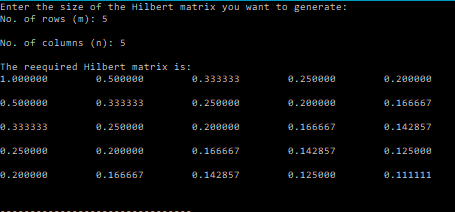# Hilbert Matrix – C PROGRAM

A Hilbert matrix is defined as:$a_{ij}=\frac{1}{i+j-1}$
where$i=1,2,3...,m$
and$j=1,2,3,....,n$

Example:$H_{2\times 2}= \begin{pmatrix} 1 & \frac{1}{2}\\ \frac{1}{2} & \frac{1}{3} \end{pmatrix}$

In this post I have written a code that generates Hilbert matrices using C language.

### CODE:

/*******************************
****HILBERT MATRIX GENERATOR****
*******************************/
#include<stdio.h>
/********
Function that generates a Hilbert matrix
Parameters:
no. of rows: m,
no. of coulmns: n,
a matrix of size mxn that would store the Hilbert matrix
********/
void Hilbert(int m, int n, double H[m][n]){
int i,j;
for(i=0;i<m;i++){
for(j=0;j<n;j++){
H[i][j]=(double)1.0/((i+1)+(j+1)-1.0);
}
}
}
/*******
Function that prints the elements of a matrix row-wise
Parameters: rows(m),columns(n),matrix[m][n]
*******/
void printMatrix(int m, int n, double matrix[m][n]){
int i,j;
for(i=0;i<m;i++){
for(j=0;j<n;j++){
printf("%lf\t",matrix[i][j]);
}
printf("\n");
}
}
int main(){
int m,n,i,j;
printf("Enter the size of the Hilbert matrix you want to generate:\nNo. of rows (m): ");
scanf("%d",&m);
printf("\nNo. of columns (n): ");
scanf("%d",&n);
double H[m][n];
Hilbert(m,n,H);
printf("\nThe reequired Hilbert matrix is:\n");
printMatrix(m,n,H);
}


### OUTPUT:### Android Apps:

I’ve also created a few Android apps that perform various matrix operations and can come in handy to those taking a course on Numerical Methods.